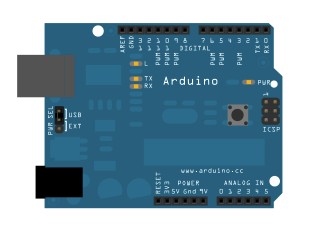Learning   Examples | Foundations | Hacking | Links

Examples > Strings

### String Comparison Operators

The String comparison operators, `==`, `!=`,`>`, `<` ,`>=`, `<=` , and the functions`equals()` and `equalsIgoreCase()` allow you to make alphabetic comparisons between Strings. They're useful for sorting and alphabetizing, among other things.

The operator `==` and the function `equals()` perform identically. It's just a matter of which you prefer. So

if (stringOne.equals(stringTwo)) {

is identical to

if (stringOne ==stringTwo) {

The greater than and less than operators evaluate strings in alphabetical order, on the first character where the two differ. So, for example `"a" < "b"` and `"1" < "2"`, but `"999"> "1000"` because 9 comes after 1.

Caution: String comparison operators can be confusing when you're comparing numeric strings, because you're used to thinking of them as numbers, not strings. If you have to compare numbers, compare them as ints, floats, or longs, and not as Strings.

### Hardware Required

• Arduino Board

### Circuit

There is no circuit for this example, though your Arduino must be connected to your computer via USB.image developed using Fritzing. For more circuit examples, see the Fritzing project page

### Code

/*
Comparing Strings

Examples of how to compare strings using the comparison operators

created 27 July 2010
modified 30 Aug 2011
by Tom Igoe

http://arduino.cc/en/Tutorial/StringComparisonOperators

This example code is in the public domain.
*/

String stringOne, stringTwo;

void setup() {
Serial.begin(9600);
stringOne = String("this");
stringTwo = String("that");
Serial.println("\n\nComparing Strings:");

}

void loop() {
// two strings equal:
if (stringOne == "this") {
Serial.println("StringOne == \"this\"");
}
// two strings not equal:
if (stringOne != stringTwo) {
Serial.println(stringOne + " =! " + stringTwo);
}

// two strings not equal (case sensitivity matters):
stringOne = "This";
stringTwo = "this";
if (stringOne != stringTwo) {
Serial.println(stringOne + " =! " + stringTwo);
}
// you can also use equals() to see if two strings are the same:
if (stringOne.equals(stringTwo)) {
Serial.println(stringOne + " equals " + stringTwo);
}
else {
Serial.println(stringOne + " does not equal " + stringTwo);
}

// or perhaps you want to ignore case:
if (stringOne.equalsIgnoreCase(stringTwo)) {
Serial.println(stringOne + " equals (ignoring case) " + stringTwo);
}
else {
Serial.println(stringOne + " does not equal (ignoring case) " + stringTwo);
}

// a numeric string compared to the number it represents:
stringOne = "1";
int numberOne = 1;
if (stringOne == numberOne) {
Serial.println(stringOne + " = " + numberOne);
}

// two numeric strings compared:
stringOne = "2";
stringTwo = "1";
if (stringOne >= stringTwo) {
Serial.println(stringOne + " >= " + stringTwo);
}

// comparison operators can be used to compare strings for alphabetic sorting too:
stringOne = String("Brown");
if (stringOne < "Charles") {
Serial.println(stringOne + " < Charles");
}

}

if (stringOne <= "Browne") {
Serial.println(stringOne + " <= Browne");
}

if (stringOne >= "Brow") {
Serial.println(stringOne + " >= Brow");
}

// the compareTo() operator also allows you to compare strings
// it evaluates on the first character that's different.
// if the first character of the string you're comparing to
// comes first in alphanumeric order, then compareTo() is greater than 0:
stringOne = "Cucumber";
stringTwo = "Cucuracha";
if (stringOne.compareTo(stringTwo) < 0 ) {
Serial.println(stringOne + " comes before " + stringTwo);
}
else {
Serial.println(stringOne + " comes after " + stringTwo);
}

delay(10000);  // because the next part is a loop:

// compareTo() is handy when you've got strings with numbers in them too:

while (true) {
stringOne = "Sensor: ";
stringTwo= "Sensor: ";